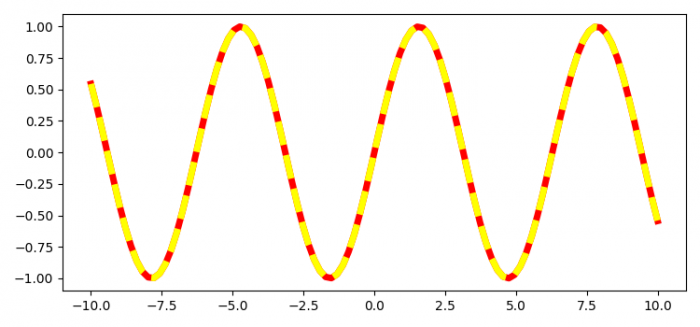# How to get alternating colours in a dashed line using Matplotlib?

To get alternating colors in a dashed line using Matplotlib, we can take the following steps −

• Set the figure size and adjust the padding between and around the subplots
• Get the current axis.
• Create x and y data points using numpy.
• Plot x and y data points with "-" and "--" linestyle.
• To display the figure, use show() method.

## Example

from matplotlib import pyplot as plt
import numpy as np

plt.rcParams["figure.figsize"] = [7.50, 3.50]
plt.rcParams["figure.autolayout"] = True

ax = plt.gca()
x = np.linspace(-10, 10, 100)
y = np.sin(x)

ax.plot(x, y, '-', color='red', linewidth=5)
ax.plot(x, y, '--', color='yellow', linewidth=5)

plt.show()

## OutputUpdated on: 10-Jun-2021

297 Views